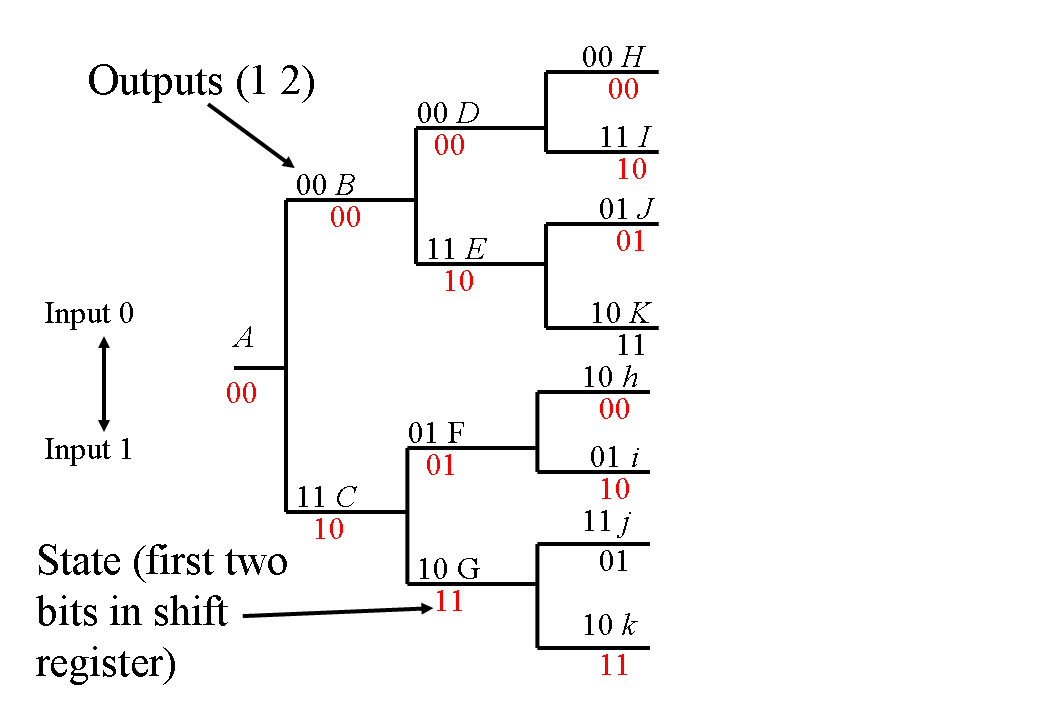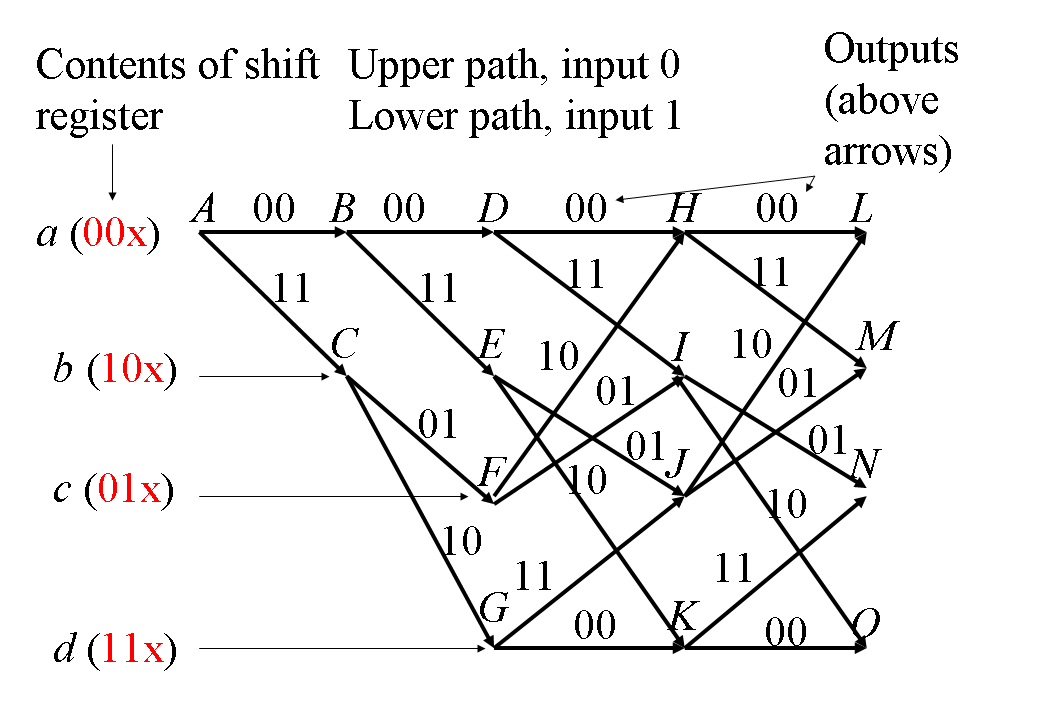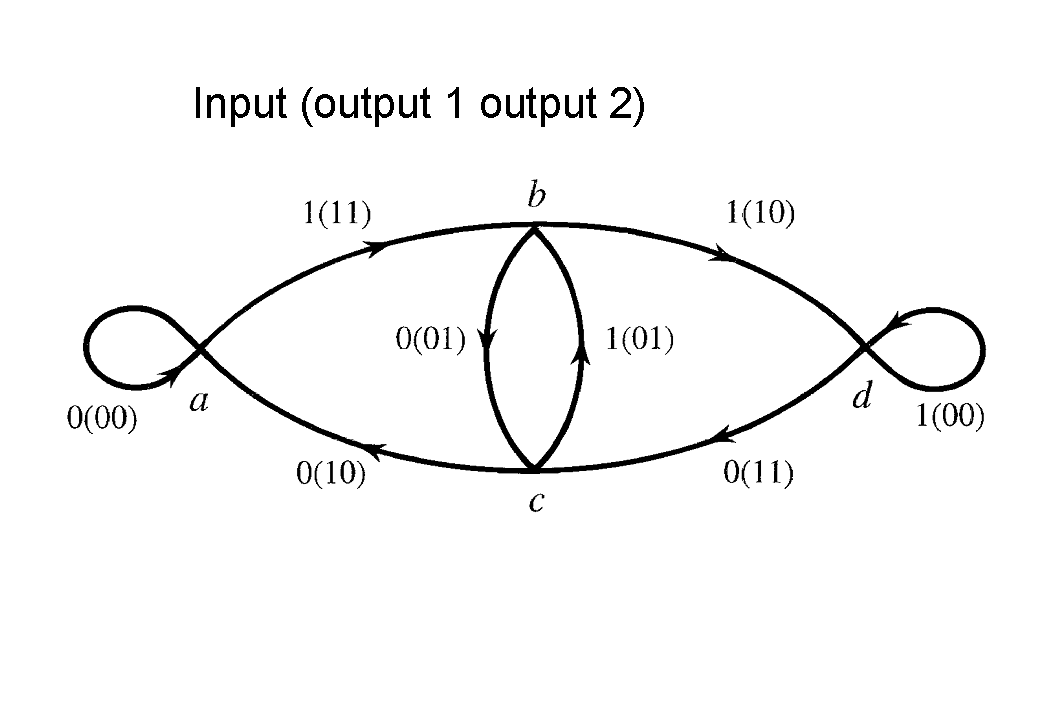0.3 Convolutional fecc encoder

 Page 1 / 1
Convolutional codes form part of forward error correcting coders (FECC). They are non-systematic and are generated by passing a data sequence through a transversal or finite impulse response (FIR) filter. This module provides an example of encoding data with a simple convolutional encoder.

Convolutional coding

Convolutional codes are another type of forward error correcting coder (FECC) which are quite distinct from block codes. They are simpler to implement for longer codes than block coders and soft decision decoding can be employed easily at the decoder.

Convolutional codes are non-systematic (i.e. the transmitted data bits do not appear directly in the output encoded data stream) and are generated by passing a data sequence through a transversal or finite impulse response (FIR) filter. The coder output can be regarded as the convolution of the input sequence with the impulse response of the coder, hence their name: convolutional codes.

Convolutional encoder

A simple example is shown in [link] . Here the encoder shift register starts with zeros at all three stored locations (i.e. 0, 0, 0). The input data sequence to be encoded is 1, 1, 0, 1 in this example. The shift register contents thus become, after each data bit arrives and propagates into the shift register: 100, 110, 011, 101. As there are two outputs for overy input bit the above encoder is rate ½.

The first output is obtained after arrival of a new data bit into the shift register when the switch is in the upper position, the second with the switch in the lower position. Thus, in this example, the switch will generate, through the exclusive OR gates, from the four input data bits: 1, 1, 0, 1, the corresponding four output digit pairs: 11, 10, 11, 01

This particular encoder has 3 stages in “the filter” and therefore we say that the constraint length n = 3. The very latest encoders available commercially typically have constraint lengths up to n = 9.

We can consider the coder outputs from the exclusive OR gates as being generated by two polynomials:

${P}_{1}\mathrm{\left(x\right)}=1+{x}^{2}$
${P}_{2}\mathrm{\left(x\right)}=1+x$

These are often expressed in octal notation, in our example:

${P}_{1}()={5}_{o}\times \left(101\right)$
${P}_{2}()={6}_{o}\times \left(110\right)$

This encoder may also be regarded as a state machine. The next state is determined by the next input bit or value combined with the previous two input bits or values which were stored in the shift register, (i.e. the previous state).

Tree state diagram

We can regard this as a Mealy state machine with four states corresponding to all the possible combinations of the first two stages in the shift register.

The tree diagram for this state machine is now shown in [link] , again starting from the all zeros state or condition. The encoder starts in state A holding two zeros (00) within the first two stages of the shift register. (We ignore the final stored digit as it is lost when a new data bit propagates into the shift register.) If the next input bit is a zero (0) we follow the upper path to state B where the stored data is updated to 00. If the next input bit is a one (1) we follow the lower path to progress to the corresponding state C where the stored data is now 10.

The convention is to enter the updated new stored state values below the state letter (B/C). Now returning to [link] and the exclusive OR gate connections one can derive the output data bits generated within the encoder. For state B these are 00 and for state C these are 11. These outputs are entered alongside the state in [link] . States B/C correspond to the arrival of the first new data bit to be encoded, while D/E/F/G correspond to the second data bit and H/I/J/K/h/i/j/k the third data bit.Encoded data tree diagram for the encoder of Figure 1

The tree diagram in [link] tends to suggest that there are eight states in the last layer of the tree and that this will continue to grow. However some states in the last layer (i.e. the stored data in the encoder) are equivalent as indicated by the same letter on the tree (for example H and h).

These pairs of states may be assumed to be equivalent because they have the same internal state for the first two stages of the shift register and therefore will behave exactly the same way to the receipt of a new (0 or 1) input data bit.

Trellis state diagram

Thus the tree can be folded into a trellis, as shown in , which is derived from the tree diagram of [link] and [link] encoder. As the constraint length is n = 3 we have ${2}^{\left(3-1\right)}$ = 4 unique states: 00, 01, 10, 11 in [link] . In [link] the states are shown as 00x to denote the third bit, x, which is lost or discarded following the arrival of a new data bit.Trellis Diagram corresponding to the Tree Diagram of Figure 2

Note in [link] the horozontal arrangement of states A, B, D, H and L. The same applies to states C, E, I and M etc. The horizontal direction corresponds to time (the whole diagram in [link] now corresponds to encoding 4 input data bits). Here we have dropped the state information from [link] as the same states are all represented at the same horizontal level in [link] . The vertical direction here corresponds to the stored state values a, b, c, d in the encoder shift register.

States along the time axis are thus equivalent, for example H is equivalent to L and C is equivalent to E etc. In fact all the states in a horizontal line are equivalent. Thus we can identify only four states in this coder: a, b, c and d and the related shift register stored values 00, 10, 01, 11 are shown in the left hand side of [link] .

From any point, e.g. E, if the next input bit is a zero (0) we follow the upper path to state J where the stored data is updated to 01 and the output will be 01. If the next input bit is a one (1) we follow the lower path from E to progress to the next state K where the stored data is now 11 and the output will be 10 as indicated alongside the trellis path.

Transition state diagram

We can draw, if desired, the trellis diagram of [link] in [link] as a state diagram containing only these states with all the corresponding new data bits to be encoded and the corresponding two output bits generated per new input data bit (e.g. 1(10))State diagram corresponding to the encoder trellis diagram of Figure 3
This module has been created from lecture notes originated by P M Grant and D G M Cruickshank which are published in I A Glover and P M Grant, "Digital Communications", Pearson Education, 2009, ISBN 978-0-273-71830-7. Powerpoint slides plus end of chapter problem examples/solutions are available for instructor use via password access at http://www.see.ed.ac.uk/~pmg/DIGICOMMS/

what is Nano technology ?
write examples of Nano molecule?
Bob
The nanotechnology is as new science, to scale nanometric
brayan
nanotechnology is the study, desing, synthesis, manipulation and application of materials and functional systems through control of matter at nanoscale
Damian
Is there any normative that regulates the use of silver nanoparticles?
what king of growth are you checking .?
Renato
What fields keep nano created devices from performing or assimulating ? Magnetic fields ? Are do they assimilate ?
why we need to study biomolecules, molecular biology in nanotechnology?
?
Kyle
yes I'm doing my masters in nanotechnology, we are being studying all these domains as well..
why?
what school?
Kyle
biomolecules are e building blocks of every organics and inorganic materials.
Joe
anyone know any internet site where one can find nanotechnology papers?
research.net
kanaga
sciencedirect big data base
Ernesto
Introduction about quantum dots in nanotechnology
what does nano mean?
nano basically means 10^(-9). nanometer is a unit to measure length.
Bharti
do you think it's worthwhile in the long term to study the effects and possibilities of nanotechnology on viral treatment?
absolutely yes
Daniel
how to know photocatalytic properties of tio2 nanoparticles...what to do now
it is a goid question and i want to know the answer as well
Maciej
characteristics of micro business
Abigail
for teaching engĺish at school how nano technology help us
Anassong
Do somebody tell me a best nano engineering book for beginners?
there is no specific books for beginners but there is book called principle of nanotechnology
NANO
what is fullerene does it is used to make bukky balls
are you nano engineer ?
s.
fullerene is a bucky ball aka Carbon 60 molecule. It was name by the architect Fuller. He design the geodesic dome. it resembles a soccer ball.
Tarell
what is the actual application of fullerenes nowadays?
Damian
That is a great question Damian. best way to answer that question is to Google it. there are hundreds of applications for buck minister fullerenes, from medical to aerospace. you can also find plenty of research papers that will give you great detail on the potential applications of fullerenes.
Tarell
what is the Synthesis, properties,and applications of carbon nano chemistry
Mostly, they use nano carbon for electronics and for materials to be strengthened.
Virgil
is Bucky paper clear?
CYNTHIA
carbon nanotubes has various application in fuel cells membrane, current research on cancer drug,and in electronics MEMS and NEMS etc
NANO
so some one know about replacing silicon atom with phosphorous in semiconductors device?
Yeah, it is a pain to say the least. You basically have to heat the substarte up to around 1000 degrees celcius then pass phosphene gas over top of it, which is explosive and toxic by the way, under very low pressure.
Harper
Do you know which machine is used to that process?
s.
how to fabricate graphene ink ?
for screen printed electrodes ?
SUYASH
What is lattice structure?
of graphene you mean?
Ebrahim
or in general
Ebrahim
in general
s.
Graphene has a hexagonal structure
tahir
On having this app for quite a bit time, Haven't realised there's a chat room in it.
Cied
how did you get the value of 2000N.What calculations are needed to arrive at it
Privacy Information Security Software Version 1.1a
Good
Got questions? Join the online conversation and get instant answers!ByBy Danielrosenberger